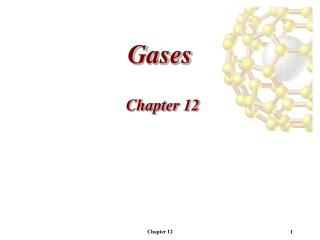DownloadDownload PresentationGases

# Gases

Télécharger la présentation## Gases

- - - - - - - - - - - - - - - - - - - - - - - - - - - E N D - - - - - - - - - - - - - - - - - - - - - - - - - - -
##### Presentation Transcript

1. Gases Chapter 12 Chapter 12

2. Characteristics of Gases • Expand to fill a volume (expandability) • Compressible • Readily forms homogeneous mixtures with other gases Chapter 12

3. Pressure Pressure - force acting on an object per unit area. Chapter 12

4. Pressure • Conversion Factors • 1 atm (atmosphere) = 760 mmHg • 1 atm (atmosphere) = 760 torr • 1 atm (atmosphere) = 1.01325  105 Pa (Pascal) • 1 atm (atmosphere) = 101.325 kPa (Kilopascal) Chapter 12

5. The Gas Laws • There are four variables required to describe a gas: • Amount of substance: moles • Volume of substance: liters • Pressures of substance: atmospheres (atm) • Temperature of substance: kelvin • The gas laws will hold two of the quantities constant and see how the other two vary. Chapter 12

6. The Gas Laws The Pressures-Volume Relationship: Boyle’s Law Boyle’s Law - The volume of a fixed quantity of gas is inversely proportional to its pressure at constant temperature. Chapter 12

7. The Gas Laws The Pressures-Volume Relationship: Boyle’s Law Boyle’s Law - The volume of a fixed quantity of gas is inversely proportional to its pressure at constant temperature. Chapter 12

8. The Gas Laws The Temperature-Volume Relationship: Charles’s Law Charles’s Law - The volume of a fixed quantity of gas at constant pressure is directly proportional to the substances temperature in Kelvin. Chapter 12

9. The Gas Laws The Temperature-Volume Relationship: Charles’s Law Charles’s Law - The volume of a fixed quantity of gas at constant pressure is directly proportional to the substances temperature in Kelvin. Chapter 12

10. The Gas Laws The General Gas Law - This is a combination of Boyle’s and Charles’s gas law. Chapter 12

11. The Gas Laws The Quantity-Volume Relationship: Avogadro’s Law Avogadro’s Law - The volume of gas at a given temperature and pressure is directly proportional to the number of moles of gas. Chapter 12

12. The Ideal Gas Equation • Combine the gas laws (Boyle, Charles, Avogadro) yields a new law or equation. Ideal gas equation: PV = nRT R = gas constant = 0.08206 L(atm)/mol(K) P = pressure (atm) V = volume (L) n = moles T = temperature (K) Chapter 12

13. The Ideal Gas Equation • We define STP (standard temperature and pressure) as 0C (273.15 K), 1 atm. • Volume of 1 mol of gas at STP is 22.4 L (molar vol.). Chapter 12

14. Applications of The Ideal-Gas Equation Gas Densities and Molar Mass • Rearranging the ideal-gas equation with M as molar mass yields Chapter 12

15. Gas Mixtures and Partial Pressures Dalton’s Law - In a gas mixture the total pressure is given by the sum of partial pressures of each component: Ptotal = P1 + P2 + P3 + … - The pressure due to an individual gas is called a partial pressure. Chapter 12

16. Gas Mixtures and Partial Pressures Partial Pressures and Mole Fractions • The partial pressure of a gas can determined if you know the mole fraction of the gas of interest and the total pressure of the system. • i is the mole fraction of gas i (ni/ntotal). Pi = iPtotal Chapter 12

17. Kinetic-Molecular Theory • Theory developed to explain gas behavior • To describe the behavior of a gas, we must first describe what a gas is: • Gases consist of a large number of molecules in constant random motion. • Volume of individual molecules negligible compared to volume of container. • Intermolecular forces (forces between gas molecules) negligible. • Energy can be transferred between molecules, but total kinetic energy is constant at constant temperature. • Average kinetic energy of molecules is proportional to temperature. Chapter 12

18. Molecular Effusion and Diffusion Graham’s Law of Effusion Graham’s Law of Effusion - The rate of effusion of a gas is inversely proportional to the square root of its molecular weight. • Effusion is the escape of a gas through a tiny hole (a balloon will deflate over time due to effusion). Chapter 12

19. Real Gases: Deviations from Ideal Behavior • The assumptions in kinetic molecular theory show where ideal gas behavior breaks down • When the volume of the gas becomes very small (the volume of the gas molecules become significant) • When the pressure become very large (gas molecules start to attract each other). Chapter 12

20. Real Gases: Deviations from Ideal Behavior The van der Waals Equation • We add two terms to the ideal gas equation one to correct for volume of molecules and the other to correct for intermolecular attractions • The correction terms generate the van der Waals equation: where a and b are empirical constants. Chapter 12

21. Homework 2, 14, 20, 28, 36, 38, 48, 56 Chapter 12